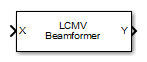# LCMV Beamformer

Narrowband linear constraint minimum variance (LCMV) beamformer

• Library:
• Phased Array System Toolbox / Beamforming

•## Description

The LCMV Beamformer block performs narrowband linear-constraint minimum-variance (LCMV) beamforming. The number of constraints must be less than the number of elements or subarrays in the array.

## Ports

### Input

expand all

Input signals to beamformer, specified as an M-by-N complex-valued matrix. M is the number of signal samples. N is the number of sensor array elements.

The size of the first dimension of the input matrix can vary to simulate a changing signal length. A size change can occur, for example, in the case of a pulse waveform with variable pulse repetition frequency.

Data Types: `double`
Complex Number Support: Yes

Training input signal, specified as a P-by-N complex-valued matrix. P is the number of samples in the training input signal. N is the number of elements of the sensor array. P must be greater than N.

The size of the first dimension of the input matrix can vary to simulate a changing signal length. A size change can occur, for example, in the case of a pulse waveform with variable pulse repetition frequency.

#### Dependencies

To enable this port, select the Enable training data input checkbox.

Data Types: `double`
Complex Number Support: Yes

### Output

expand all

Beamformed output, specified as an M-by-1 complex-valued column vector. M is the number of signal samples.

Data Types: `double`
Complex Number Support: Yes

Beamformer weights output, specified as an N-by-1 complex-valued column vector. N is the number of array elements.

#### Dependencies

To enable this port, select the Enable weights output checkbox.

Data Types: `double`
Complex Number Support: Yes

## Parameters

expand all

LCMV beamformer constraint matrix specified as an N-by-K complex-valued matrix. Each column of the matrix is a constraint. N is the number of elements in the sensor array and K is the number of constraints. K must be less than or equal to the number of sensors, N,K ≤ N

Desired response of the LCMV beamformer, specified as a real-valued K-by-1 column vector. K is the number of constraints in the Constraint matrix. Each element in the vector defines the desired response of the constraint specified in the corresponding column of the Constraint matrix parameter.

Diagonal loading factor, specified as a positive scalar. Diagonal loading is a technique used to achieve robust beamforming performance, especially when the sample support is small.

Enable training data input port, specified as `off` or `on`. To enable the training data input port, `XT`, select this checkbox.

Enable beamforming weights output port, specified as `off` or `on`. To enable the beamforming weights output port, `W`, select this checkbox.

Block simulation, specified as `Interpreted Execution` or `Code Generation`. If you want your block to use the MATLAB® interpreter, choose `Interpreted Execution`. If you want your block to run as compiled code, choose ```Code Generation```. Compiled code requires time to compile but usually runs faster.

Interpreted execution is useful when you are developing and tuning a model. The block runs the underlying System object™ in MATLAB. You can change and execute your model quickly. When you are satisfied with your results, you can then run the block using ```Code Generation```. Long simulations run faster with generated code than in interpreted execution. You can run repeated executions without recompiling, but if you change any block parameters, then the block automatically recompiles before execution.

This table shows how the Simulate using parameter affects the overall simulation behavior.

When the Simulink® model is in `Accelerator` mode, the block mode specified using Simulate using overrides the simulation mode.

Acceleration Modes

 Block Simulation Simulation Behavior `Normal` `Accelerator` `Rapid Accelerator` `Interpreted Execution` The block executes using the MATLAB interpreter. The block executes using the MATLAB interpreter. Creates a standalone executable from the model. `Code Generation` The block is compiled. All blocks in the model are compiled.